# The "Einstein-Laue" discussion

### By Wilfried Schroeder and Hans-Juergen Treder

In this note we consider the historical and theoretical origin of relativistic thermodynamics in the recent theory of black-body radiation in relation to a recent paper by Liu1.

1. The Planck-Einstein transformation of temperature

In 1952 Einstein turned from the Planck-Einstein transformation formulas (1907) to the heat Q and therefore the temperature T. Instead of the transformation laws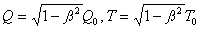(where ss = v/c)

(which were generally accepted at the time), Einstein suggested the transformations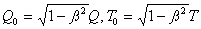These are the laws which H. Ott2 proposed some years later (1963), and independently of Einstein´s results. C. Lui emphasizes that Einstein´s second letter to Laue (1953) implies universal scepticism with respect to the memory of the relativistic thermodynamics which were acutalized in the following decades3.

The key problem is the comparison of the socalled “eigen.temperature” of two bodies which are in motion relative to each other and the heat transport between bodies with variable distance and stressed heat conductor.

From a historical standpoint, the primary idea in the works of K. von Mosengeil4 and M Planck5 was the measurement of the black radiation temperature of moving bodies. In this case, we do not have the problem of heat transport between bodies. A system of black bodies at relative rest, which is in a state of radiation equilibrium, remains in this state if the bodies are diabatically accelerated to each other.

If, in the rest state, the “eigen-temperature” of the bodies is T0, then the “eigen-temperature” of the moving bodies become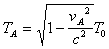where vA is the velocity of a body PA relative to the rest system6.

This axiom is a result of Kirchhoff´s theorem and is based on the validity of Wien´s law in each reference system. Wien´s law implies that the variable n / T (where n is the frequency) in the Kirchhoff-Planck radiation function is Lorentz-invariant. The the Planck-Einstein transformation of the black-body temperature T follows from Einstein´s theory of relativistic Doppler effect and abberation7: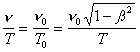The Planck-Einstein formulation of relativistic thermodynamics is primarly the thermodynamics of heat radiation and involves the definition of the temperature of bodies according to their black-body radiation8.

2. Historical remarks on Planck´s relativistic thermodynamics

Planck´s relativistic thermodynamics was originally a thermodynamics of black-body radiation or heat radiation (Planck´s “Hohlraum-Strahlung”). In 1906, Planck published his book on heat radiation9. It contained a definiton of thermodynamic equilibrium according to Kirchhoff´s law and introduced an “entropy radiation”10.

Planck´s first approach to relativistic thermodynamics was based on the thesis of his former pupil Kurt von Mosengeil. Max von Laue pointed out the importance of this publication11. Von Mosengeil´s transformation of heat, energy, pressure and volume V became a special case of Planck´s notation. If the pressure p is the Maxwellian radiation pressure, P = 2u, and if the radiation density is u = aT4 according to the Stefan-Boltzmann law12, Von Mosengeil´s transformation of the black radiation entropy13.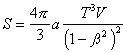(where a is Stefan´s constant)

gives, according to the Lorentz contraction, V – (1-ss2)0,5 V0 and with respect to Planck´s theorem of the invariance of entropy, S = S0, the temperature transformation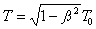.

1. C. Liu: Einstein and relativistic thermodynamics in 1952: a historical and critical study of a strange episode in the history of modern physics. BJHS (1992), 25, 185-206
2. H. Ott: Lorentz-Transformationen der Waerme und der Temperature. Z. Phys. (1963), 175, 70-104
3. Cf. the discussion in R. G. Newburgh: Relativistic thermodynamics, temperature, transformations, invariance and measurements. Nuovo Cimente (1979) B52, 219-28
4. K. von Mosengeil: Theorie der stationaeren Strahlung in einem gleichfoermig bewegten Hohlraum. Ann. Phys. (1907), 22, 876-906
5. M. Planck: Zur Dynamik bewegter Systeme. Berliner Berichte (1908), 542-70
6. A. Einstein: Ueber das Relativitaetsprinzip und die aus demselben gezogenen Folgerungen. Jhb. Radioakt. Elektron.
(1907), 4, 411-62
7. Ibid.
8. H.-J. Treder: Die Strahlungstemperatur bewegter Koerper. Ann. Phy. (1977), 34, 23-9
9. M. Planck: Theorie der Waermestrahlung, Leipzig (Bahrt), 1906
10. Ibid.
11. M. von Laue: Relativitaetsprinzip und Wien´sches Gesetz. Ann. Phys. (1943), 34, 220-2
12. Von Mosengeil, op. cit.
(4), 876-904
13. The Problem of von Mosengeil´s thesis was a critical revision and correction to an (non-relativistic) paper by F. Hasenroehrl: Zur Theorie der Strahlung bewegter Koerper. Ann. Phys. (1904), 45, 344-70. M. Abraham: Elektromagnetische Theorie der Strahlung (§44, Dynamik des bewegten Hohlraums), Leipzig, 1920; see also W. Pauli: Relativitaetstheorie, Enzyklop. Math. Wiss., Leipzig, 1921, xix (cf. §49a: Schwarze Strahlung in einem bewegte Hohlraum).